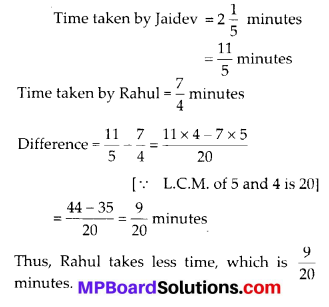# MP Board Class 6th Maths Solutions Chapter 7 Fractions Ex 7.6

## MP Board Class 6th Maths Solutions Chapter 7 Fractions Ex 7.6

Question 1.
Solve:Solution: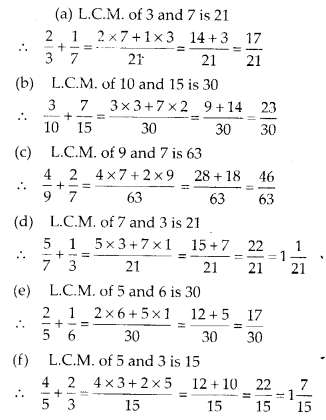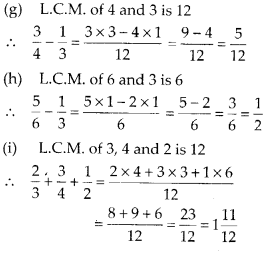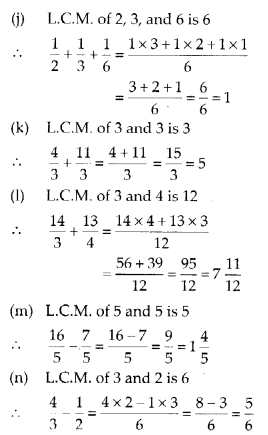Question 2.
Sarita bought $$\frac{2}{5}$$ metre of ribbon and Lalita $$\frac{3}{4}$$ metre of ribbon. What is the total length of the
ribbon they bought?
Solution:
Ribbon bought by Sarita = $$\frac{2}{5}$$ m
And Ribbon bought by Lalita = $$\frac{3}{4}$$ m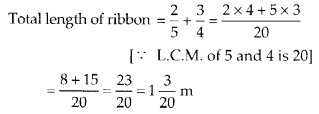Therefore, they bought 1$$\frac{3}{20}$$ m of ribbon.Question 3.
Naina was given 1$$\frac{1}{22}$$ piece of cake and Najma was given 1$$\frac{1}{3}$$ piece of cake. Find the total amount of cake was given to both of them.
Solution:
Cake taken by Naina = 1$$\frac{1}{2}$$ piece
And cake taken by Najma = 1$$\frac{1}{3}$$ piece
Total cake taken = $$1 \frac{1}{2}+1 \frac{1}{3}=\frac{3}{2}+\frac{4}{3}$$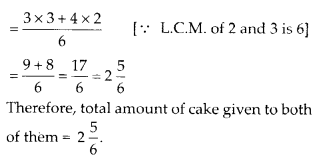Question 4.
Fill in the boxes: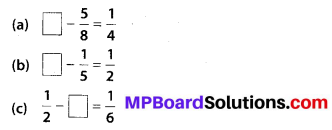Solution: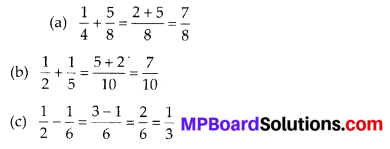Question 5.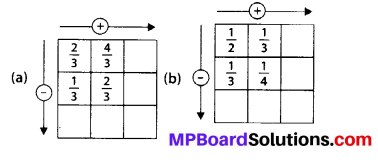Solution: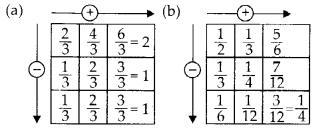Question 6.
A piece of wire $$\frac{7}{8}$$ metre long broke into two pieces. One piece was $$\frac{1}{4}$$ metre long. How long is the other piece?
Solution:
Total length of wire = $$\frac{7}{8}$$ metre
Length of first part = $$\frac{1}{4}$$ metre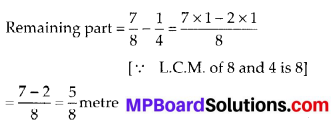Therefore, the length of remaining part is $$\frac{5}{8}$$ metre

Question 7.
Nandini’s house is $$\frac{9}{10}$$ metre km from her school. She 10
walked some distance and then took a bus for $$\frac{1}{2}$$ metre km to reach the school. How far did she walk?
Solution:
Total distance between school and house = $$\frac{9}{10}$$ km
Distance covered bv bus = $$\frac{1}{2}$$ kmTherefore, distance covered by walking is $$\frac{2}{5}$$ km.Question 8.
Asha and Samuel have bookshelves of the same size partly filled with books. Asha’s shelf is $$\frac{5}{6}$$ th full and Samuel’s shelf is $$\frac{2}{5}$$th full. Whose bookshelf is more full? By what fraction?
Solution: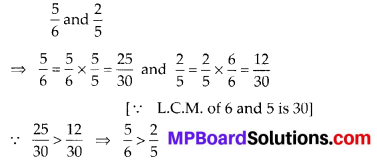∴ Asha’s bookshelf is more covered than Samuel.
Difference $$=\frac{25}{30}-\frac{12}{30}=\frac{13}{30}$$Question 9.
Jaidev takes 2$$\frac{1}{5}$$ minutes to walk across the school ground. Rahul takes $$\frac{7}{4}$$ minutes to do the same. Who takes less time and by what fraction?
Solution: